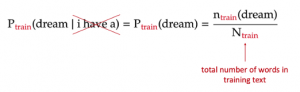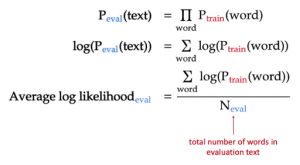# N-gram language models – Part 1Estimated probability of the unigram ‘dream’ from the training text

## Evaluating the model

After estimating all unigram probabilities, we can apply these estimates to calculate the probability of each sentence in the evaluation text: each sentence probability is the product of word probabilities.

We can go further than this and estimate the probability of the entire evaluation text, such as dev1 or dev2. Under the naive assumption that each sentence in the text is independent of other sentences, we can decompose this probability as the product of the sentence probabilities, which in turn are nothing but products of word probabilities.

## The role of ending symbols

As outlined above, our language model not only assigns probabilities to words but also probabilities to all sentences in a text. As a result, to ensure that the probabilities of all possible sentences sum to 1, we need to add the symbol `[END]` to the end of each sentence and estimate its probability as if it is a real word. This is a rather esoteric detail, and you can read more about its rationale here (page 4).

## Evaluation metric: average log-likelihood

When we take the log on both sides of the above equation for the probability of the evaluation text, the log probability of the text (also called log-likelihood), becomes the sum of the log probabilities for each word. Lastly, we divide this log-likelihood by the number of words in the evaluation text to ensure that our metric does not depend on the number of words in the text.For n-gram models, log of base 2 is often used due to its link to information theory (see here, page 21)

As a result, we end up with the metric of average log-likelihood, which is simply the average of the trained log probabilities of each word in our evaluation text. In other words, the better our language model is, the probability that it assigns to each word in the evaluation text will be higher on average.

Other common evaluation metrics for language models include cross-entropy and perplexity. However, they still refer to the same thing: cross-entropy is the negative of average log-likelihood, while perplexity is the exponential of cross-entropy.

Please check more detail from the Link

Please check Gaussian Sample and Bayesian Statistics.

# Hiring Data Scientist / Engineer

We are looking for Data Scientist and Engineer.
Please check our Career Page.

# Data Science Project

Please check about experiences for Data Science Project

# Vietnam AI / Data Science LabPlease also visit Vietnam AI Lab